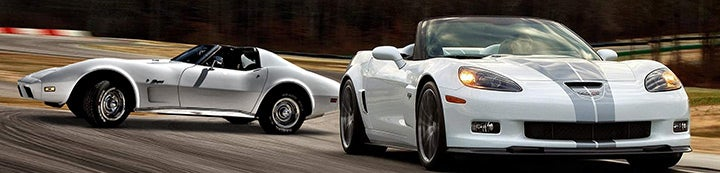1 - 6 of 6 Posts

Santacruz

·
Registered
Joined
·
136 Posts
Discussion Starter · ·
I had a link saved on my old computer that I no longer have that showed how to read a VIN number. Does anyone have the chart for this?

Uncle Tom

·
Registered
Joined
·
195 Posts
Last 6 digits. Subtract 100000 from the last 6 digits.

This is an example of a VIN # for a 2006 Corvette Coupe:
1 G 1 Y Y 2 6 U 3 6 5 1 0 0 0 0 1
There are 17 characters. Starting with first character on left the codes are:
1. "1"= Built in US
2. "G"= General Motors
3. "1"= Chevrolet
4. Denotes the car line: Corvette is a "Y" body.
Usually a "Y" but the ZR-1 is "Z"
6. Body style: "1" = FRC, "2" = Coupe, "3" = Convertible
7. Restraint code
8. Engine:
L98="8"
LT1="P"
ZR1="J"
LT4="5"
LS1="G"
LS6="S”
LS2=”U”
LS3=”W”
LS7=”E”
9. Check Digit – 0 to 9 or X
10. Model year:
"B" thru "N" are yrs '81-'92 respectively
"P"='93
"R" thru "T" '94-'96
"V"='97
"W"='98
"X"='99
"Y"='00
"1"='01
“5”=’05
“6”=’06
“7”=’07
11. Plant: Bowling Green = "5"
12-17. Production sequence number that starts with the #1 for each model yr.

Capt Kirk

·
Registered
Joined
·
221 Posts
Last 6 digits. Subtract 100000 from the last 6 digits.

This is an example of a VIN # for a 2006 Corvette Coupe:
1 G 1 Y Y 2 6 U 3 6 5 1 0 0 0 0 1
There are 17 characters. Starting with first character on left the codes are:
1. "1"= Built in US
2. "G"= General Motors
3. "1"= Chevrolet
4. Denotes the car line: Corvette is a "Y" body.
Usually a "Y" but the ZR-1 is "Z"
6. Body style: "1" = FRC, "2" = Coupe, "3" = Convertible
7. Restraint code
8. Engine:
L98="8"
LT1="P"
ZR1="J"
LT4="5"
LS1="G"
LS6="S”
LS2=”U”
LS3=”W”
LS7=”E”
9. Check Digit – 0 to 9 or X
10. Model year:
"B" thru "N" are yrs '81-'92 respectively
"P"='93
"R" thru "T" '94-'96
"V"='97
"W"='98
"X"='99
"Y"='00
"1"='01
“5”=’05
“6”=’06
“7”=’07
11. Plant: Bowling Green = "5"
12-17. Production sequence number that starts with the #1 for each model yr.

:thumbsup3: :thumbsup3:

justflewby

·
Registered
Joined
·
143 Posts
Last 6 digits. Subtract 100000 from the last 6 digits.

This is an example of a VIN # for a 2006 Corvette Coupe:
1 G 1 Y Y 2 6 U 3 6 5 1 0 0 0 0 1
There are 17 characters. Starting with first character on left the codes are:
1. "1"= Built in US
2. "G"= General Motors
3. "1"= Chevrolet
4. Denotes the car line: Corvette is a "Y" body.
Usually a "Y" but the ZR-1 is "Z"
6. Body style: "1" = FRC, "2" = Coupe, "3" = Convertible
7. Restraint code
8. Engine:
L98="8"
LT1="P"
ZR1="J"
LT4="5"
LS1="G"
LS6="S”
LS2=”U”
LS3=”W”
LS7=”E”
9. Check Digit – 0 to 9 or X
10. Model year:
"B" thru "N" are yrs '81-'92 respectively
"P"='93
"R" thru "T" '94-'96
"V"='97
"W"='98
"X"='99
"Y"='00
"1"='01
“5”=’05
“6”=’06
“7”=’07
11. Plant: Bowling Green = "5"
12-17. Production sequence number that starts with the #1 for each model yr.
:goodpost:

JamesC6

·
Registered
Joined
·
7 Posts
Cool! Printing out, I have a folder in the garage with quick reference documents that I have found over the years.

juicyj

·
Registered
Joined
·
3 Posts
Look at the first number displayed on your Corvette's VIN number to discover its country of origin.

Read the second character on the Corvette's VIN number. This will be a letter "G" which stands for General Motors.

Look at characters 4 and 5 to determine your Corvette's make. The fourth character is a "Y" for Y-body series

Read character number 6 to determine a Corvette's body style. A number "1" means that it is a two-door fixed-top coupe (hard top), a number "2" signifies that it is a two-door hatchback and a number "3" stands for two-door convertible.

Look at the seventh character. This will be a number 2 that means active belts with front air bag, or a number 4 that stands for active belts with front and side air bag.

Look at character number 10 to determine your Corvette's year. This function started in 1981, and each year was represented alphabetically with a different letter.

Look at the 11th digit to identify the name of the production plant where your Corvette was assembled.

But as for me, everything can be done much easier and just enter the VIN code of your car into this VIN Decoder
Thus, you can find out all the necessary information about the car. Hope I helped you!

1 - 6 of 6 Posts